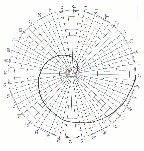Archimedian Spirals

Archimedian Spirals Constructed on Polargraphic Paper with Geometric AlgebraSingle Turn Spiral This is the trajectory of a point that travels .27... rads (unit of distance from center) every 10° of rotation from 0 to 360° , going 10 rads in the process from the center to the outside, making one turn of the spiral for the 360° rotation. This constant increase in the distance from the center, lets call it Delta rad or D(r), here is .027.../degree, or .27... rads/ 10° counter clockwise rotation we used in this construction. Such a constant increase defines an Archimedian spiral, after the Greek mathematician, Archimedes, who studied them extensively. Thus, the total rads, R(n), at any step n, equals the previous total, R(n-1) plus D(r), or R(n) = R(n-1) + D(r) = n * D(r)   In this case of the one turn spiral, R(n) = 36 * .277 where n=36, the total number of 10° steps taken from the initial position at 0°. Thus after the 36 steps to complete the circle, 36 * .27...rads = 10 rads.Created: 11/24/97

Updated: 12/7/97